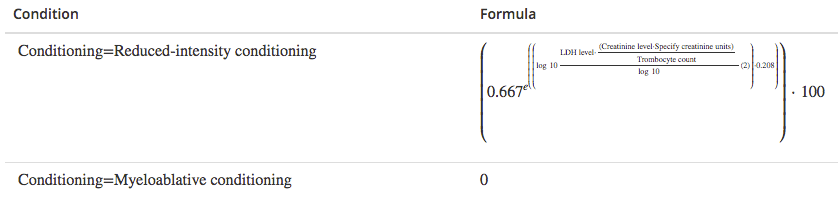Evidencio Calculations

We want to be fully transparent regarding the underlying calculations for the standard model types that Evidencio supports. This page lists our standard model types and the associated calculations

Linear Regression Models

Linear regression models are used to predict continuous outcomes. The prediction is estimated by:
predicted result P = \beta_0 + X\beta

Logistic Regression Models:

Logistic regression models are used to predict probabilities. The prediction is estimated by:
predicted probability P = e^(X\beta)/(1+e^(X\beta))

Cox proportional-hazards regression

Cox proportional-hazards models are used to predict the probability S(t) that the patient will be free from an event (e.g. biochemical recurrence) at some time point (e.g. 5 years after surgery). For survival analysis the prediction is estimated by: S(t) = S_0(t)^exp(X\beta)

Custom Models

Users can define Custom prediction models based on custom mathematical formulas expressed in terms of model variables. Evidencio supports both single and conditional custom formulas. The custom formula(s) used by an Evidencio model can always be viewed in the details section of the respective model, so that model users can exactly identify the underlying model logic.
Example of conditional formulas:R-Script Models

Evidencio supports the use of R-Script code to define the calculation logic for prediction models and calculators. R-Script models may contain complex and nested functions as long as they produce a single value outcome.

Parameter transformations

Evidencio lets you define variable/parameter transformations for each continuous model variable, for instance to accommodate non linear behaviour. We support both simple transformations as well as conditional (range based) transformations (e.g. cubic splines) to tackle a broad spectrum of non-linear behaviour.

Confidence interval data

You can attribute confidence interval data to your models on Evidencio. Your confidence interval data can be specified in terms of a bootstrap data-set or as covariance matrix. You can simply copy paste your bootstrap or matrix data from e.g. excel or another data-sheet.

Calculation Legend
 \beta_0 Intercept term, denoted by "Intercept" \beta_(varN) Coefficient estimate, associated with a covariate/variable \gamma Scaling parameter in survival regression models, denoted by "Scaling Parameter" X\beta Model linear predictor, estimated by summation of the intercept term and all \beta values multiplied by that variable. E.g.: \beta_0 + \beta_(var1) * var1 + \beta_(var2) * var2 + \beta_(varN) * varN S_0(t) The baseline survival function, the survival proportion when all covariates are equal to zero (X\beta = 0).